• 2020-06-18 09:43:49

什么是深层信仰网络？

深度信念网络是使用概率和无监督学习来产生输出的算法。它们由二进制潜在变量组成，并且包含无向层和有向层。

与其他模型不同，深度信任网络中的每一层都学习整个输入。在卷积神经网络中，第一层仅过滤基本特征（例如边缘）的输入，而第二层则重组前一层找到的所有简单模式。另一方面，深度信仰网络在全局范围内运作，并按顺序调节每一层。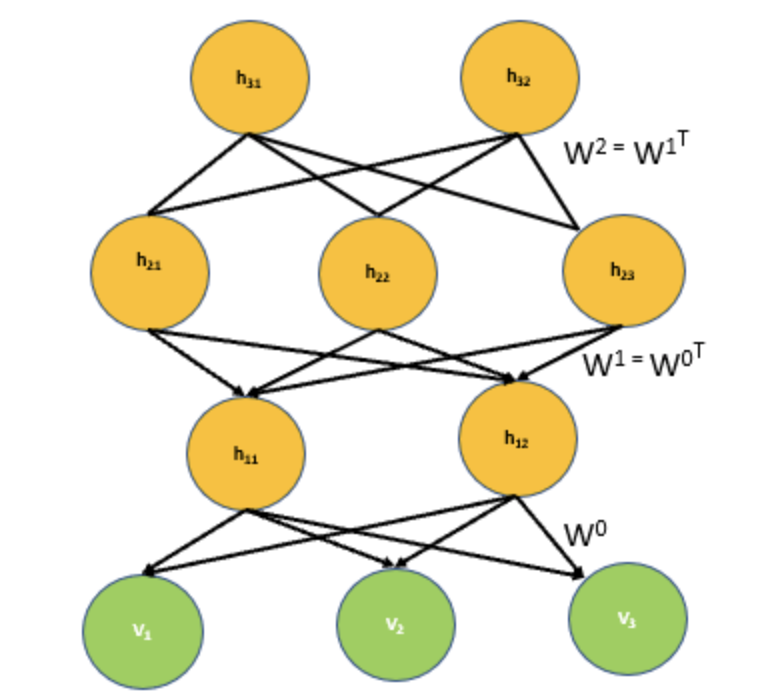深度信任网络（DBN）架构

网络就像一堆受限的玻尔兹曼机器（RBM），其中每层中的节点都连接到上一层和下一层中的所有节点。但是，与RBM不同，深度信任网络中的节点不在其层内进行横向通信。对称权重的网络连接不同的层。

顶层中的连接是无向的，并且由它们之间的连接形成关联存储器。较低级别的连接是定向的。

隐藏层中的节点扮演两个角色-它们充当其之前节点的隐藏层，并充当其后节点的可见层。这些节点标识数据中的相关性。

深度信仰网络如何工作？

贪婪学习算法用于预训练深度信念网络。这是一个解决问题的方法，涉及在序列中的每一层进行最优选择，最终找到全局最优值。

贪婪的学习算法从底层开始，然后向上移动，以微调生成权重。该学习是逐层进行的，这意味着深度信念网络的各层一次被训练。因此，每一层还接收不同版本的数据，并且每一层都使用前一层的输出作为输入。

贪婪学习算法可用于训练深度信任网络，因为它们快速有效。此外，它们有助于优化每一层的权重。

深度信念网络的应用

影像识别

深度信念网络可用于图像识别。图片将是输入，类别将是输出。这项技术具有广泛的应用，范围从相对简单的任务（如照片整理）到关键功能（如医学诊断）。例如，可以执行图像识别的智能小孢子可以用来对病原体进行分类。这将减轻严重流行病期间对稀有专家的依赖，减少响应时间。

视频识别

视频识别还使用深度信任网络。视频识别与视觉类似，因为它可以在视频数据中找到含义。例如，它可以识别物体或人的手势。它可以用于许多不同的领域，例如家庭自动化，安全性和医疗保健。

运动捕捉数据

运动捕获数据涉及跟踪对象或人的运动，并且还使用深度信任网络。运动捕捉非常棘手，因为一台机器可能会很快失去对某个人的跟踪，例如，如果另一个看上去相似的人进入帧，或者某物暂时遮挡了他们的视线。因此，运动捕捉不仅取决于物体或人的外观，而且还取决于速度和距离。运动捕捉广泛用于视频游戏开发和电影制作中。

深度信念神经网络如何演变？
第一代神经网络使用的感知器通过考虑“weight”或来识别特定物体或其他物体。但是，感知器只能在基本级别上有效，而对先进技术则无济于事。为了解决这些问题，第二代神经网络引入了反向传播的概念，其中将接收到的输出与所需的输出进行比较，并将误差值减小为零。支持向量机通过参考先前输入的测试用例来创建和理解更多的测试用例。接下来是一个称为信念网络的循环图，该循环图有助于解决与推理和学习问题有关的问题。随后是Deep Belief Networks，它帮助创建了要存储在叶节点中的无偏值。

受限玻尔兹曼机
深度信任网络由无监督的网络（如RBM）组成。在此，每个子网的不可见层是下一个子网的可见层。隐藏或不可见的层彼此不连接，并且在条件上是独立的。在所有可见层和隐藏层上建立联合配置网络的可能性取决于联合配置网络的能量与所有其他联合配置网络的能量相比。

训练深层置信网络
第一步是训练一层可以直接从像素获得输入信号的属性。下一步是将该层的值视为像素，并在第二个隐藏层中学习先前获得的特征。每次将另一层属性或特征添加到置信网络时，训练数据集的对数概率的下限将得到改善。算法
更多相关内容
• 为了设计一种适合大规模复制的低成本解决方案，本文提出了一种数据驱动的方法通过使用即时深度信任网络（JIT-DBN）来填充错误控制。 该方法旨在基于历史数据构建本地DBN模型，通过综合考虑流体粘度，填充温度和许多...
• 为了解决这个问题，本文提出了一种新的半监督学习算法，称为深度信念网络，嵌入了Softmax回归算法（DBNESR）。 DBNESR首先通过深度学习来学习特征的层次表示，然后使用Softmax回归进行更有效的分类。 同时，我们...
• 深度信念网络是一个生成模型，用来生成符合特定分布的样本。隐变量用来描述在可观测变量之间的高阶相关性。假如加入服从分布 ()的训练数据，通过训练得到一个深度信念网络。 生成样本时，先在最顶两层进行足够多的...

# 三、DBN

## 3.1 生成模型

深度信念网络是一个生成模型，用来生成符合特定分布的样本。隐变量用来描述在可观测变量之间的高阶相关性。假如加入服从分布 𝑝(𝑣)的训练数据，通过训练得到一个深度信念网络。生成样本时，先在最顶两层进行足够多的吉布斯采样，在达到热平衡时生成样本ℎ^((𝐿−1))，然后依次计算下一层隐变量的分布。因为在给定上一层变量取值时，下一层的变量是条件独立的，故可独立采样。这样，从第𝐿−1层开始，自顶向下进行逐层采样，最终得到可观测层的样本。

## 3.2 参数学习

深度信念网络最直接的训练方式是最大化可观测变量的边际分布𝑝(𝑣)在训练集上的似然 。但是在深度信念网络中，隐变量ℎ之间的关系十分复杂，由于“贡献度分配问题”，很难直接学习。即使对于简单的单层Sigmoid信念网络：

p ( v = 1 ∣ h ) = σ ( b + ω T h ) p(v=1 \mid h)=\sigma\left(b+\omega^{T} h\right)

在已知可观测变量时，其隐变量的联合后验概率𝑝(ℎ|𝑣)不再相互独立，因此很难估计所有隐变量的后验概率，早期深度信念网络的后验概率一般通过蒙特卡洛方法或变分方法来近似估计，但效率低，从而导致其参数学习比较困难。

为了有效训练深度信念网络，我们将每一层的Sigmoid信念网络转换为受限玻尔兹曼机，这样做的好处是隐变量的后验概率事相互独立的，从而可容易进行采样。这样，深度信念网络可看做多个受限玻尔兹曼机从下到上进行堆叠，第𝑙层受限玻尔兹曼机的隐层作为第𝑙+1受限玻尔兹曼机的可观测层。进一步，深度信念网络可采用逐层训练的方式来快速训练，即从最底层开始，每次只训练一层，直到最后一层。

深度信念网络的训练过程可分为逐层预训练和精调两个阶段，先通过逐层预训练将模型的参数初始化为较优的值，然后通过传统机器学习方法对参数进行精调。

## 3.2 逐层预训练

采用逐层训练的方式，将深度信念网络的训练简化为对多个受限玻尔兹曼机的训练。具体的逐层训练过程为自下而上依次训练每一层的首先玻尔兹曼机。假设已训练好前𝑙−1层的受限玻尔兹曼机，可计算隐变量自下而上的条件概率：

p ( h ( i ) ∣ h ( i − 1 ) ) = σ ( b i + W ( i ) h ( i − 1 ) ) , 1 ≤ i ≤ ( l − 1 ) p\left(h^{(i)} \mid h^{(i-1)}\right)=\sigma\left(b^{i}+W^{(i)} h^{(i-1)}\right), 1 \leq i \leq(l-1)

这样可按照 𝑣 = h ( 0 ) → ⋯ → h ( 𝑙 − 1 ) 𝑣=ℎ^{(0)}→⋯→ℎ^{(𝑙−1)} 的顺序生成一组 h 𝑙 − 1 ℎ^{𝑙−1} 的样本，记为 H ( l − 1 ) = h ( l , 1 ) , . . . , h ( l , M ) H^{(l-1)} = h^{(l,1)},...,h^{(l,M)} 。然后将 h ( 𝑙 − 1 ) ℎ^{(𝑙−1)} h ( 𝑙 ) ℎ^{(𝑙)} 组成一个受限玻尔兹曼机，用 𝐻 ( 𝑙 − 1 ) 𝐻^{(𝑙−1)} 作为训练集充分训练第𝑙层的受限玻尔兹曼机.大量实践表明，逐层预训练可以产生非常好的参数初始值，从而极大地降低了模型的学习难度。

## 3.3 精调

经过预训练，再结合具体的任务（监督或无监督学习），通过传统的全局学习算法对网络进行精调（fine-tuning），使模型收敛到更好的局部最优点。

作为生成模型的精调：除了顶层的受限玻尔兹曼机，其它层之间的权重可以被分为向上的认知权重（Recognition Weight） 𝑊 ∗ 𝑊^* 和向下的生成权重（Generative Weight）𝑊。认知权重用来计算后验概率，生成权重用来定义模型，认知权重初始值 𝑊(‘𝑙)=𝑊(𝑙)𝑇

深度信念网络一般采用 Contrastive Wake-Sleep算法进行精调：

1.Wake阶段：认知过程，通过外界输入（可观测变量）和向上的认知权重，计算每一层隐变量的后验概率并采样。修改下行的生成权重使得下一层的变量的后验概率最大。

2.Sleep阶段：生成过程，通过顶层的采样和向下的生成权重，逐层计算每一层的后验概率并采样。然后，修改向上的认知权重使得上一层变量的后验概率最大。

3.交替进行Wake和Sleep过程，直到收敛作为判别模型的精调 ：深度信念网络的一个应用是作为深度神经网络的预训练模型，提供神经网络的初始权重，这时只需要向上的认知权重，作为判别模型使用：# 四 代码演示

import sys
import numpy

numpy.seterr(all='ignore')

def sigmoid(x):
return 1. / (1 + numpy.exp(-x))

def softmax(x):
e = numpy.exp(x - numpy.max(x))  # prevent overflow
if e.ndim == 1:
return e / numpy.sum(e, axis=0)
else:
return e / numpy.array([numpy.sum(e, axis=1)]).T  # ndim = 2

class DBN(object):
def __init__(self, input=None, label=None,\
n_ins=2, hidden_layer_sizes=[3, 3], n_outs=2,\
numpy_rng=None):

self.x = input
self.y = label

self.sigmoid_layers = []
self.rbm_layers = []
self.n_layers = len(hidden_layer_sizes)  # = len(self.rbm_layers)

if numpy_rng is None:
numpy_rng = numpy.random.RandomState(1234)

assert self.n_layers > 0

# construct multi-layer
for i in range(self.n_layers):
# layer_size
if i == 0:
input_size = n_ins
else:
input_size = hidden_layer_sizes[i - 1]

# layer_input
if i == 0:
layer_input = self.x
else:
layer_input = self.sigmoid_layers[-1].sample_h_given_v()
# print('=============')

# construct sigmoid_layer
sigmoid_layer = HiddenLayer(input=layer_input,
n_in=input_size,
n_out=hidden_layer_sizes[i],
numpy_rng=numpy_rng,
activation=sigmoid)
self.sigmoid_layers.append(sigmoid_layer)

# construct rbm_layer
rbm_layer = RBM(input=layer_input,
n_visible=input_size,
n_hidden=hidden_layer_sizes[i],
W=sigmoid_layer.W,     # W, b are shared
hbias=sigmoid_layer.b)
self.rbm_layers.append(rbm_layer)

# layer for output using Logistic Regression
self.log_layer = LogisticRegression(input=self.sigmoid_layers[-1].sample_h_given_v(),
label=self.y,
n_in=hidden_layer_sizes[-1],
n_out=n_outs)

# finetune cost: the negative log likelihood of the logistic regression layer
self.finetune_cost = self.log_layer.negative_log_likelihood()

def pretrain(self, lr=0.1, k=1, epochs=100):
# pre-train layer-wise
# print('pre-training')
for i in range(self.n_layers):
if i == 0:
layer_input = self.x
else:
layer_input = self.sigmoid_layers[i-1].sample_h_given_v(layer_input)
# print(layer_input)
rbm = self.rbm_layers[i]

for epoch in range(epochs):
rbm.contrastive_divergence(lr=lr, k=k, input=layer_input)
# cost = rbm.get_reconstruction_cross_entropy()
# print >> sys.stderr, \
#        'Pre-training layer %d, epoch %d, cost ' %(i, epoch), cost

# def pretrain(self, lr=0.1, k=1, epochs=100):
#     # pre-train layer-wise
#     for i in range(self.n_layers):
#         rbm = self.rbm_layers[i]

#         for epoch in range(epochs):
#             layer_input = self.x
#             for j in range(i):
#                 layer_input = self.sigmoid_layers[j].sample_h_given_v(layer_input)

#             rbm.contrastive_divergence(lr=lr, k=k, input=layer_input)
#             # cost = rbm.get_reconstruction_cross_entropy()
#             # print >> sys.stderr, \
#             #        'Pre-training layer %d, epoch %d, cost ' %(i, epoch), cost

def finetune(self, lr=0.1, epochs=100):
# print('finetune')
layer_input = self.sigmoid_layers[-1].sample_h_given_v()

# train log_layer
epoch = 0
done_looping = False
while (epoch < epochs) and (not done_looping):
self.log_layer.train(lr=lr, input=layer_input)
# self.finetune_cost = self.log_layer.negative_log_likelihood()
# print >> sys.stderr, 'Training epoch %d, cost is ' % epoch, self.finetune_cost

lr *= 0.95
epoch += 1

def predict(self, x):
layer_input = x

for i in range(self.n_layers):
sigmoid_layer = self.sigmoid_layers[i]
# rbm_layer = self.rbm_layers[i]
layer_input = sigmoid_layer.output(input=layer_input)

out = self.log_layer.predict(layer_input)
return out

class HiddenLayer(object):
def __init__(self, input, n_in, n_out,\
W=None, b=None, numpy_rng=None, activation=numpy.tanh):

if numpy_rng is None:
numpy_rng = numpy.random.RandomState(1234)

if W is None:
a = 1. / n_in
initial_W = numpy.array(numpy_rng.uniform(  # initialize W uniformly
low=-a,
high=a,
size=(n_in, n_out)))

W = initial_W

if b is None:
b = numpy.zeros(n_out)  # initialize bias 0

self.numpy_rng = numpy_rng
self.input = input
self.W = W
self.b = b

self.activation = activation

def output(self, input=None):
if input is not None:
self.input = input

linear_output = numpy.dot(self.input, self.W) + self.b

return (linear_output if self.activation is None
else self.activation(linear_output))

def sample_h_given_v(self, input=None):
if input is not None:
self.input = input

v_mean = self.output()
# print('v_mean:\n',v_mean)
h_sample = self.numpy_rng.binomial(size=v_mean.shape,
n=1,
p=v_mean)
# print('h_sample:\n',h_sample)
return h_sample

class RBM(object):
def __init__(self, input=None, n_visible=2, n_hidden=3, \
W=None, hbias=None, vbias=None, numpy_rng=None):

self.n_visible = n_visible  # num of units in visible (input) layer
self.n_hidden = n_hidden    # num of units in hidden layer

if numpy_rng is None:
numpy_rng = numpy.random.RandomState(1234) # 使用RandomState获得随机数生成器。

if W is None:
a = 1. / n_visible
initial_W = numpy.array(numpy_rng.uniform(  # initialize W uniformly
low=-a,
high=a,
size=(n_visible, n_hidden)))  # 生成权重矩阵

W = initial_W

if hbias is None:
hbias = numpy.zeros(n_hidden)  # initialize h bias 0

if vbias is None:
vbias = numpy.zeros(n_visible)  # initialize v bias 0

self.numpy_rng = numpy_rng
self.input = input
self.W = W
self.hbias = hbias
self.vbias = vbias
# self.params = [self.W, self.hbias, self.vbias]

def contrastive_divergence(self, lr=0.1, k=1, input=None):
if input is not None:
self.input = input

''' CD-k '''
ph_mean, ph_sample = self.sample_h_given_v(self.input)

chain_start = ph_sample

for step in range(k):
if step == 0:
nv_means, nv_samples,\
nh_means, nh_samples = self.gibbs_hvh(chain_start)
else:
nv_means, nv_samples,\
nh_means, nh_samples = self.gibbs_hvh(nh_samples)
# nv_means, nv_samples, nh_means, nh_samples = self.gibbs_hvh(chain_start)
# for _ in range(1, k):
#         nv_means, nv_samples, nh_means, nh_samples = self.gibbs_hvh(nh_samples)

# chain_end = nv_samples

self.W += lr * (numpy.dot(self.input.T, ph_sample)
- numpy.dot(nv_samples.T, nh_means))
self.vbias += lr * numpy.mean(self.input - nv_samples, axis=0)
self.hbias += lr * numpy.mean(ph_sample - nh_means, axis=0)

# cost = self.get_reconstruction_cross_entropy()
# return cost

def sample_h_given_v(self, v0_sample):
h1_mean = self.propup(v0_sample)  #
h1_sample = self.numpy_rng.binomial(size=h1_mean.shape,   # discrete: binomial 二项分布
n=1,
p=h1_mean)

return [h1_mean, h1_sample]

def sample_v_given_h(self, h0_sample):
v1_mean = self.propdown(h0_sample)
v1_sample = self.numpy_rng.binomial(size=v1_mean.shape,   # discrete: binomial
n=1,
p=v1_mean)
return [v1_mean, v1_sample]

def propup(self, v):
# 返回隐藏层被激活的概率，应该是一个矩阵，对应的是每一个神经元被激活的概率
pre_sigmoid_activation = numpy.dot(v, self.W) + self.hbias
return sigmoid(pre_sigmoid_activation)

def propdown(self, h):
pre_sigmoid_activation = numpy.dot(h, self.W.T) + self.vbias
return sigmoid(pre_sigmoid_activation)

def gibbs_hvh(self, h0_sample):
v1_mean, v1_sample = self.sample_v_given_h(h0_sample)
h1_mean, h1_sample = self.sample_h_given_v(v1_sample)
return [v1_mean, v1_sample, h1_mean, h1_sample]

def get_reconstruction_cross_entropy(self):
pre_sigmoid_activation_h = numpy.dot(self.input, self.W) + self.hbias
sigmoid_activation_h = sigmoid(pre_sigmoid_activation_h)

pre_sigmoid_activation_v = numpy.dot(sigmoid_activation_h, self.W.T) + self.vbias
sigmoid_activation_v = sigmoid(pre_sigmoid_activation_v)

cross_entropy =  - numpy.mean(
numpy.sum(self.input * numpy.log(sigmoid_activation_v) +
(1 - self.input) * numpy.log(1 - sigmoid_activation_v),
axis=1))

return cross_entropy

def reconstruct(self, v):
h = sigmoid(numpy.dot(v, self.W) + self.hbias)
reconstructed_v = sigmoid(numpy.dot(h, self.W.T) + self.vbias)
return reconstructed_v

class LogisticRegression(object):
def __init__(self, input, label, n_in, n_out):
self.x = input
self.y = label
self.W = numpy.zeros((n_in, n_out))  # initialize W 0
self.b = numpy.zeros(n_out)          # initialize bias 0

def train(self, lr=0.1, input=None, L2_reg=0.00):
if input is not None:
self.x = input

p_y_given_x = softmax(numpy.dot(self.x, self.W) + self.b)
d_y = self.y - p_y_given_x

self.W += lr * numpy.dot(self.x.T, d_y) - lr * L2_reg * self.W
self.b += lr * numpy.mean(d_y, axis=0)

def negative_log_likelihood(self):
sigmoid_activation = softmax(numpy.dot(self.x, self.W) + self.b)

cross_entropy = - numpy.mean(
numpy.sum(self.y * numpy.log(sigmoid_activation) +
(1 - self.y) * numpy.log(1 - sigmoid_activation),
axis=1))

return cross_entropy

def predict(self, x):
return softmax(numpy.dot(x, self.W) + self.b)

def test_dbn(pretrain_lr=0.1, pretraining_epochs=1000, k=1, \
finetune_lr=0.1, finetune_epochs=200):

x = numpy.array([[1,1,1,0,0,0],
[1,0,1,0,0,0],
[1,1,1,0,0,0],
[0,0,1,1,1,0],
[0,0,1,1,0,0],
[0,0,1,1,1,0]])
y = numpy.array([[1, 0],
[1, 0],
[1, 0],
[0, 1],
[0, 1],
[0, 1]])

rng = numpy.random.RandomState(123)

# construct DBN
# print('construct DBN')
dbn = DBN(input=x, label=y, n_ins=6, hidden_layer_sizes=[3, 3], n_outs=2, numpy_rng=rng)

# pre-training (TrainUnsupervisedDBN)
# k是gibbs的次数

dbn.pretrain(lr=pretrain_lr, k=1, epochs=pretraining_epochs)

# fine-tuning (DBNSupervisedFineTuning)
dbn.finetune(lr=finetune_lr, epochs=finetune_epochs)

# test
x = numpy.array([1, 1, 0, 0, 0, 0])
print(dbn.predict(x))

if __name__ == "__main__":
test_dbn()

[0.72344411 0.27655589]



展开全文机器学习
• ## DBN深度信念网络详解

万次阅读 多人点赞 2018-09-11 15:44:24
自联想神经网络是很古老的神经网络模型，简单的说，它就是三层BP网络，只不过它的输出等于输入。很多时候我们并不要求输出精确的等于输入，而是允许一定的误差存在。所以，我们说，输出是对输入的一种重构。其网络...

## 1.  自联想神经网络与深度网络

自联想神经网络是很古老的神经网络模型，简单的说，它就是三层BP网络，只不过它的输出等于输入。很多时候我们并不要求输出精确的等于输入，而是允许一定的误差存在。所以，我们说，输出是对输入的一种重构。其网络结构可以很简单的表示如下：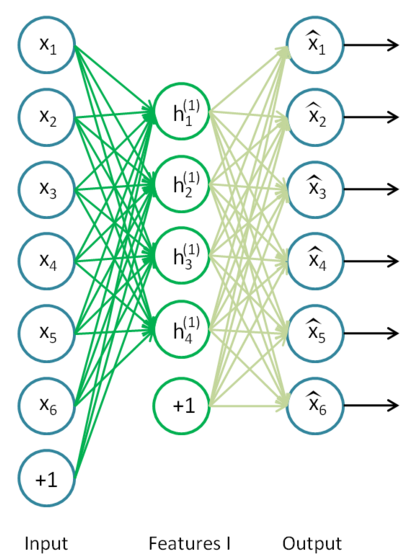如果我们在上述网络中不使用sigmoid函数，而使用线性函数，这就是PCA模型。中间网络节点个数就是PCA模型中的主分量个数。不用担心学习算法会收敛到局部最优，因为线性BP网络有唯一的极小值。

在深度学习的术语中，上述结构被称作自编码神经网络。从历史的角度看，自编码神经网络是几十年前的事情，没有什么新奇的地方。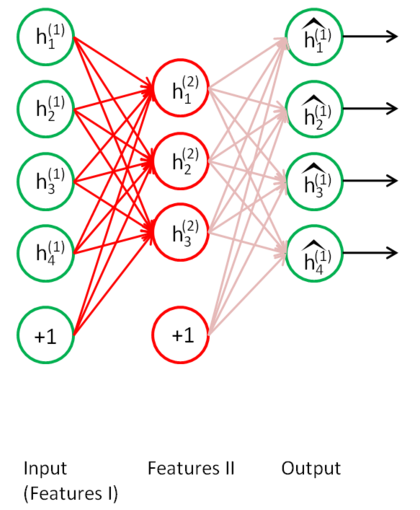既然自联想神经网络能够实现对输入数据的重构，如果这个网络结构已经训练好了，那么其中间层，就可以看过是对原始输入数据的某种特征表示。如果我们把它的第三层去掉，这样就是一个两层的网络。如果，我们把这个学习到特征再用同样的方法创建一个自联想的三层BP网络，如上图所示。换言之，第二次创建的三层自联想网络的输入是上一个网络的中间层的输出。用同样的训练算法，对第二个自联想网络进行学习。那么，第二个自联想网络的中间层是对其输入的某种特征表示。如果我们按照这种方法，依次创建很多这样的由自联想网络组成的网络结构，这就是深度神经网络，如下图所示：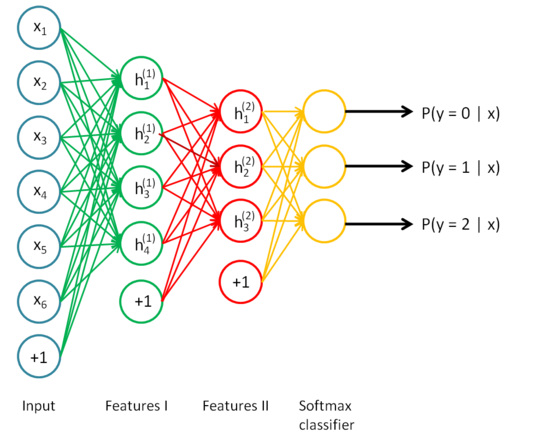注意，上图中组成深度网络的最后一层是级联了一个softmax分类器。

深度神经网络在每一层是对最原始输入数据在不同概念的粒度表示，也就是不同级别的特征描述。    这种层叠多个自联想网络的方法，最早被Hinton想到了。从上面的描述中，可以看出，深度网络是分层训练的，包括最后一层的分类器也是单独训练的，最后一层分类器可以换成任何一种分类器，例如SVM，HMM等。上面的每一层单独训练使用的都是BP算法。 相信这一思路，Hinton早就实验过了。

## 2. DBN神经网络模型

使用BP算法单独训练每一层的时候，我们发现，必须丢掉网络的第三层，才能级联自联想神经网络。然而，有一种更好的神经网络模型，这就是受限玻尔兹曼机。使用层叠波尔兹曼机组成深度神经网络的方法，在深度学习里被称作深度信念网络DBN，，这是目前非常流行的方法。下面的术语，将把自联想网络称作自编码网络autoencoder.。通过层叠自编码网络的深度网络在深度学习里另外一个属于叫栈式自编码网络。经典的DBN网络结构 是由若干层 RBM 和一层 BP 组成的一种深层神经网络, 结构如下图所示.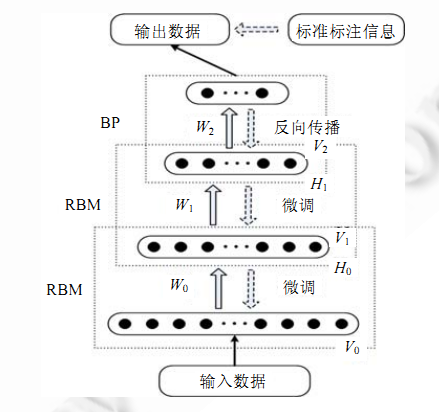DBN 在训练模型的过程中主要分为两步:

第 1 步：分别单独无监督地训练每一层 RBM 网络,确保特征向量映射到不同特征空间时,都尽可能多地保留特征信息;(学习特征)

第 2 步：在 DBN 的最后一层设置 BP 网络,接收 RBM 的输出特征向量作为它的输入特征向量,有监督地训练实体关系分类器.而且每一层 RBM 网络只能确保自身层内的 权值对该层特征向量映射达到最优,并不是对整个 DBN 的特征向量映射达到最优,所以反向传播网络还将错误信息自顶向下传播至每一层 RBM,微调整个 DBN 网络.RBM 网络训练模型的过程可以看作对一个深层 BP 网络权值参数的初始化,使DBN 克服了 BP 网络因随机初始化权值参数而容易陷入局部最优和训练时间长的缺点。

上述训练模型中第一步在深度学习的术语叫做预训练，第二步叫做微调。最上面有监督学习的那一层，根据具体的应用领域可以换成任何分类器模型，而不必是BP网络。

## 3. 深度信念网络的应用

由于自编码网络可以对原始数据在不同概念的粒度上进行抽象，深度网络一种自然的应用是对数据进行压缩或者叫降维。胡邵华等，他们用一种自编码网络实现了对经典的"瑞士卷"数据的重构: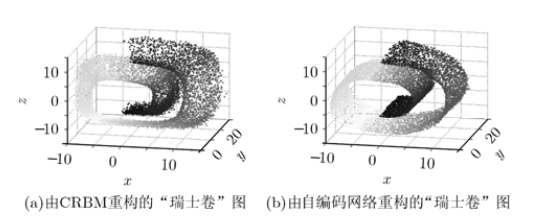" 瑞士卷"数据是经典的机器学习中难于分类的数据之一，其隐含的数据内在模式难以在二维数据中描述。然而， 胡邵华等，采用深度信念网络实现了对三维瑞士卷数据的2维表示，其自编码网络节点大小依次为3-100-50-25-10-2. 具体的实现细节请参考文献：胡邵华、宋耀良：基于autoencoder网络的数据降维与重构。

深度神经网络的另一个常见的应用是特征提取。

文献：Philippe Hamel and Douglas Eck, LEARNING FEATURES FROM MUSIC AUDIO WITH DEEP BELIEF NETWORKS.

通过训练一个5层的深度网络提取音乐的特征，用于音乐风格的分类，其分类精度比基于梅尔倒谱系数特征分类的方法提到了14个百分点。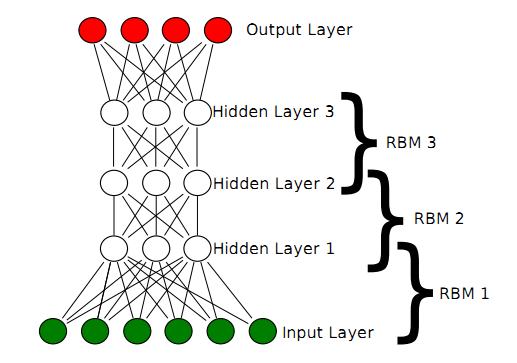他们的实现思路非常简单，用上述层叠的多个RBM网络组成深度网络结构来提取音乐的特征。输入的原始数据是经过分帧，加窗之后的信号的频谱。分类器采用的是支撑矢量机SVM。对比的方法则是提取MFCC特征系数，分类器同样采用SVM。更多的细节和实验结果可以参考上面提到的文献。

深度网络是一种良好的无监督学习方法，其特征提取功能能够针对不同概念的粒度大小，能够在很多领域得到广泛的应用。通常，DBN主要用于对一维数据的建模比较有效，例如语音。而通过级联多层卷积网络组成深度网络的模型主要用于二维数据，例如图像等。

通过下面的图以及上面的内容，可以更加深入的理解DBN：深度信念网络算法。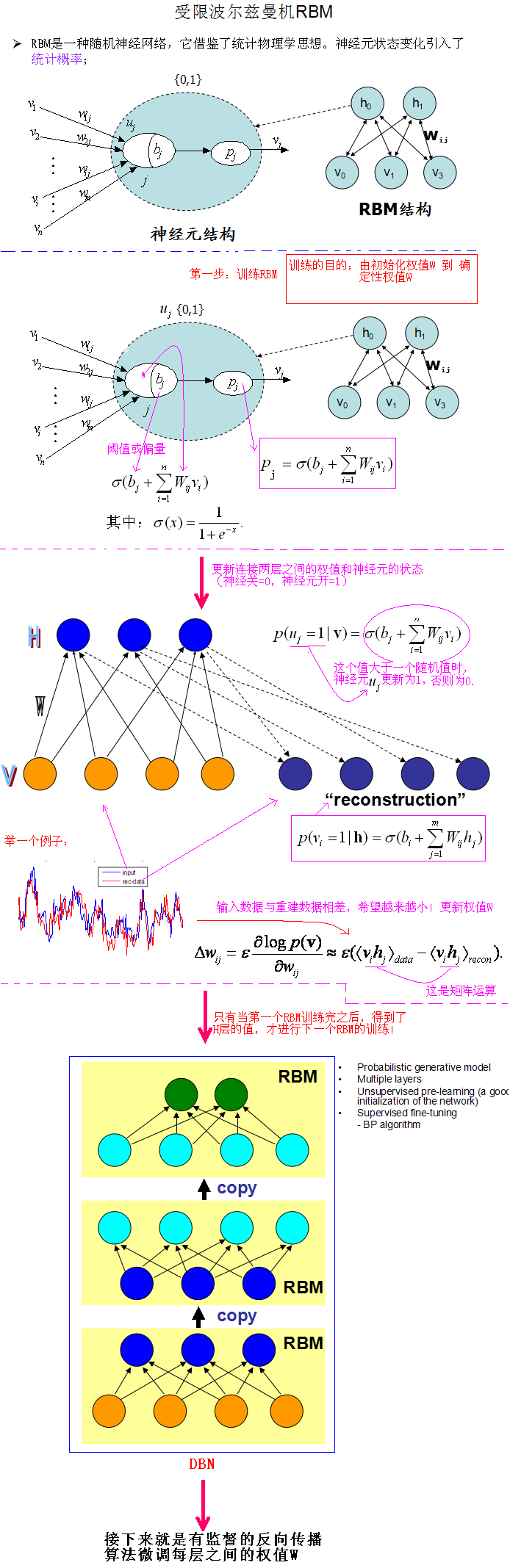参考文献：
Hinton G E, Salakhutdinov R R. Reducing the dimensionality of data with neural networks. Science, vol. 313, pp. 504-507, 2006.
Hinton G E, Osindero S, Teh Y W. A fast learning algorithm for deep belief nets. Neural computation, vol. 18, pp. 1527-1554, 2006.
Xie, Jipeng, et al. "Learning features from High Speed Train vibration signals with Deep Belief Networks." Neural Networks (IJCNN), 2014 International Joint Conference on. IEEE, 2014.
Bengio Y, Lamblin P, Popovici D, et al. Greedy layer-wise training of deep networks. Advances in neural information processing systems, vol. 19, pp. 153-160, 2007.
Salakhutdinov R. Learning deep generative models. Diss. University of Toronto, 2009.
Hinton G. A practical guide to training restricted Boltzmann machines. Neural Networks: Tricks of the Trade, pp. 599-619, 2012.
Bengio Y. Learning deep architectures for AI. Foundations and trends® in Machine Learning, vol. 2, pp. 1-127, 2009.

http://blog.csdn.net/celerychen2009/article/details/9079715

展开全文• 本篇非常简要地介绍了深度信念网络的基本概念。文章先简要介绍了深度信念网络（包括其应用实例）。接着分别讲述了：(1) 其基本组成结构——受限玻尔兹曼机的的基本情况，以及，(2) 这个基本结构如何组成深度信念网络...
本篇非常简要地介绍了深度信念网络的基本概念。文章先简要介绍了深度信念网络（包括其应用实例）。接着分别讲述了：(1) 其基本组成结构——受限玻尔兹曼机的的基本情况，以及，(2) 这个基本结构如何组成深度信念网络。 本文仅仅能使读者了解深度信念网络这一概念，内容非常浅显，甚至有许多不严密的地方。如果有愿意深入了解受限玻尔兹曼机、深度信念网络的，想对深度学习有更多了解的，请访问深度学习官方网站。或者读者可以参考文末的“推荐阅读”一节。

目录
概述 .............................................................................................................................. 2
受限玻尔兹曼机 ............................................................................................................ 3
神经元之间的条件独立性 .......................................................................................... 3
使用 RBM 的过程 ..................................................................................................... 4
训练 RBM ................................................................................................................. 5
深度信念网络 ................................................................................................................ 5
训练过程： ................................................................................................................ 6
调优过程 (Fine-Tuning) ： ...................................................................................... 6
使用过程 ................................................................................................................... 7
推荐阅读 ....................................................................................................................... 8
论文 .......................................................................................................................... 8
博文和网络教程 ........................................................................................................ 8

概述
深度信念网络 (Deep Belief Network, DBN) 由 Geoffrey Hinton 在 2006 年提出。它是一种生成模型，通过训练其神经元间的权重，我们可以让整个神经网络按照最大概率来生成训练数据。我们不仅可以使用 DBN 识别特征、分类数据，还可以用它来生成数据。下面的图片展示的是用 DBN 识别手写数字：图 1 用深度信念网络识别手写数字。图中右下角是待识别数字的黑白位图，它的上方有三层隐性神经元。每一个黑色矩形代表一层神经元，白点代表处于开启状态的神经元，黑色代表处于关闭状态的神经元。注意顶层神经元的左下方即使别结果，与画面左上角的对应表比对，得知这个 DBN 正确地识别了该数字。

下面是展示了一个学习了大量英文维基百科文章的 DBN 所生成的自然语言段落：
In 1974 Northern Denver had been overshadowed by CNL, and several Irish intelligence agencies in the Mediterranean region. However, on the Victoria, Kings Hebrew stated that Charles decided to escape during an
alliance. The mansion house was completed in 1882, the second in its bridge are omitted, while closing is the proton reticulum composed below it aims, such that it is the blurring of appearing on any well-paid type of box printer.

DBN 由多层神经元构成，这些神经元又分为 显性神经元隐性神经元（以下简称显元和隐元）。显元用于接受输入，隐元用于提取特征。因此隐元也有个别名，叫 特征检测器 (feature detectors)。最顶上的两层间的连接是无向的，组成 联合内存 (associative memory)。较低的其他层之间有连接上下的有向连接。最底层代表了 数据向量 (data vectors)，每一个神经元代表数据向量的一维。
DBN 的组成元件是 受限玻尔兹曼机 (Restricted Boltzmann Machines, RBM)。训练 DBN 的过程是一层一层地进行的。在每一层中，用数据向量来推断隐层，再把这一隐层当作下一层 (高一层) 的数据向量。

受限玻尔兹曼机
如前所述，RBM 是 DBN 的组成元件。事实上，每一个 RBM 都可以单独用作聚类器。
RBM 只有两层神经元，一层叫做 显层 (visible layer)，由 显元 (visible units) 组成，用于输入训练数据。另一层叫做 隐层 (Hidden layer)，相应地，由 隐元 (hidden units) 组成，用作 特征检测器 (feature detectors)。图 2 受限玻尔兹曼机的结构。图中的较上一层神经元组成隐层，较下的神经元组成显元。每一层都可以用一个向量来表示，每一维表示每个神经元。注意这两层间的对称 (双向) 连接。

神经元之间的条件独立性
应注意到，显层和隐层内部的神经元都没有互连，只有层间的神经元有对称的连接线。这样的好处是，在给定所有显元的值的情况下，每一个隐元取什么值是互不相关的。也就是说，同样，在给定隐层时，所有显元的取值也互不相关：有了这个重要性质，我们在计算每个神经元的取值情况时就不必每次计算一个，而是同时并行地计算整层神经元。

使用 RBM 的过程
假设我们现在已经得到一个训练好的 RBM，每个隐元与显元间的权重用矩阵
W表示，且：其中Wij代表从第i个显元到第j个隐元的权重，M代表显元的个数，N代表隐元的个数。那么，当我们把一条新来的数据附给 (clamp to) 显层后，RBM 将会依照权值W来决定开启或关闭隐元。具体的操作如下：
首先，将每个隐元的激励值 (activation) 计算出来：注意，这里用到了前面提到的神经元之间的条件独立性。
然后，将每个隐元的激励值都用 S 形函数进行标准化，变成它们处于开启状 (用 1 表示) 的概率值：此处的 S 形函数我们采用的是 Logistic 函数：至此，每个隐元hj开启的概率被计算出来了。其处于关闭状态 (用 0 表示) 的概率自然也就是那么到底这个元开启还是关闭，我们需要将开启的概率与一个从 0, 1 均匀分布中抽取的随机值进行如下比较然后开启或关闭相应的隐元。
给定隐层，计算显层的方法是一样的。

训练 RBM
RBM 的训练过程，实际上是求出一个最能产生训练样本的概率分布。也就是说，要求一个分布，在这个分布里，训练样本的概率最大。由于这个分布的决定性因素在于权值W ，所以我们训练 RBM 的目标就是寻找最佳的权值。为了保持读者的兴趣，这里我们不给出最大化对数似然函数的推导过程，直接说明如何训练 RBM。
G. Hinton 提出了名为对比散度 (Contrastive Divergence) 的学习算法。下面我们来详述它的具体过程。
我们沿用前文的符号记法。
算法1. 对比散度
对于训练集中的每一条记录如此训练之后的 RBM 就能较为准确地提取显层的特征，或者根据隐层所代表
的特征还原显层了。

深度信念网络
前文我们已经介绍了 RBM 的基本结构和其训练、使用过程，接下来我们介绍DBN 的相关内容。
DBN 是由多层 RBM 组成的一个神经网络，它既可以被看作一个生成模型，也可以当作判别模型，其训练过程是：使用非监督贪婪逐层方法去预训练获得权值。
训练过程：
1. 首先充分训练第一个 RBM；
2. 固定第一个 RBM 的权重和偏移量，然后使用其隐性神经元的状态，作为第二个 RBM 的输入向量；
3. 充分训练第二个 RBM 后，将第二个 RBM 堆叠在第一个 RBM 的上方；
4. 重复以上三个步骤任意多次；
5. 如果训练集中的数据有标签，那么在顶层的 RBM 训练时，这个 RBM 的显层中除了显性神经元，还需要有代表分类标签的神经元，一起进行训练：
a) 假设顶层 RBM 的显层有 500 个显性神经元，训练数据的分类一共分成了 10 类；
b) 那么顶层 RBM 的显层有 510 个显性神经元，对每一训练训练数据，相应的标签神经元被打开设为 1，而其他的则被关闭设为 0。
6. DBN 被训练好后如下图： (示意)图 3 训练好的深度信念网络。图中的绿色部分就是在最顶层 RBM 中参与训练的标签。注意调优 (FINE-TUNING) 过程是一个判别模型
另：调优过程 (Fine-Tuning) ：
生成模型使用 Contrastive Wake-Sleep 算法进行调优，其算法过程是：
1. 除了顶层 RBM，其他层 RBM 的权重被分成向上的认知权重和向下的生成权重；
2. Wake 阶段：认知过程，通过外界的特征和向上的权重 (认知权重) 产生每一层的抽象表示 (结点状态) ，并且使用梯度下降修改层间的下行权重 (生成权重) 。也就是“如果现实跟我想象的不一样，改变我的权重使得我想
象的东西就是这样的”。
3. Sleep 阶段：生成过程，通过顶层表示 (醒时学得的概念) 和向下权重，生成底层的状态，同时修改层间向上的权重。也就是“如果梦中的景象不是我脑中的相应概念，改变我的认知权重使得这种景象在我看来就是这个概
念”。

使用过程 ：
1. 使用随机隐性神经元状态值，在顶层 RBM 中进行足够多次的吉布斯抽样；
2. 向下传播，得到每层的状态。

推荐阅读
论文
1. Representation Learning: A Review and New Perspectives, Yoshua Bengio, Aaron Courville, Pascal Vincent, Arxiv, 2012.
2. The monograph or review paper Learning Deep Architectures for AI (Foundations & Trends in Machine Learning, 2009).
3. Deep Machine Learning – A New Frontier in Artificial Intelligence Research – a survey paper by Itamar Arel, Derek C. Rose, and Thomas P. Karnowski.
4. A Fast Learning Algorithm for Deep Belief Nets by Geoffrey E. Hinton and Simon Osindero.
博文和网络教程
1. Introduction to Restricted Boltzmann Machines by Edwin Chen.
2. An Introduction to Restricted Boltzmann Machines by Yuhuan Jiang.
3. Restricted Boltzmann Machine - Short Tutorial by iMonad.
4. 《深度学习学习笔记整理系列》 by Zouxy.
展开全文• 要运行模型，请： 1) Download the MNIST dataset (four files: t10k-images.idx3-ubyte, t10k-labels.idx1-ubyte, train-images.idx3-ubyte, train-labels.idx1-ubyte) from Yann LeCun's Website ...
• 云环境下基于BP神经网络的电子商务企业信任评估模型研究.pdf
• 文章先简要介绍了深度信念网络（包括其应用实例）。接着分别讲述了：(1) 其基本组成结构——受限玻尔兹曼机的的基本情况，以及，(2) 这个基本结构如何组成深度信念网络。 本文仅仅能使读者了解深度信念网络这...
• 基于BP神经网络模型的移动电子商务客户信任评价.pdf
• ‍‍作者： 北邮 GAMMA Lab 硕士生 刘洪瑞，副教授 王啸1 前言深度学习在计算机视觉、自然语言处理、数据挖掘等诸多研究领域中的潜力被广泛认可，在目标检测、语音识别、医疗检测、金融...算法 计算机视觉 机器学习 人工智能
• ## 深度信念网络（DBN）

万次阅读 多人点赞 2016-12-13 12:47:20
1.初识深度信念网络　深度信念网络是一个概率生成模型，与传统的判别模型的神经网络相对，生成模型是建立一个观察数据和标签之间的联合分布，对P(Observation|Label)和 P(Label|Observation)都做了评估，而判别模型...
• 也没啥原创，就是在学习深度学习的过程中丰富一下我的博客，嘿嘿。 不喜勿喷！ Hinton是深度学习方面的大牛，跟着大牛走一般不会错吧…… 来源：A fast learning algorithm for deep ...
• matlab信任模型代码基于社交的推荐网络中的项目评分预测 在这项研究中，我们的目标是在给定两个训练数据集的情况下，预测用户对某项产品的评分。 评分数据集。 信任数据集。 我们证明，简单的深层前馈神经网络...
• 企业信息化管理的战略框架与成熟度模型 现代企业架构框架白皮书 信息安全研究框架 企业数字化转型成熟度模型IOMM ...网络空间的信任模型 研发运维一体化（DevOps）成熟度模型 云计算的安全框架 智慧城市安全体系框架
• 一、复杂度与Big-O 标记 ...【2】10篇必读深度图像修复文章，珍贵老照片有救了！：https://zhuanlan.zhihu.com/p/337095820 【3】AI深度学习修复老照片--教程：https://www.bilibili.com/video/av797567017 ...python
• 然后描述了三种典型的深度学习模型，包括卷积神经网络模型、深度信任网络模型和堆栈自编码网络模型，并对近几年深度学习在初始化方法、网络层数和激活函数的选择、模型结构、学习算法和实际应用这四个方面的研究新...堆栈
• 在过去五年中，算法的重大进步很大程度上是由一类特定的模型（称为深度神经网络）推动的2。DNN是由多个处理层组成的计算模型，每一层都能够学习越来越抽象的、与执行特定任务相关的输入数据的高级表示。它们极大地...算法
• 人工智能是追求目标，机器学习是实现手段，深度学习是其中一种方法。 1 机器学习 机器学习是一类算法的总称，这些算法企图从大量历史数据中挖掘出其中隐含的规律，并用于预测或者分类，更具体的说，机器学习可以...算法 python
• 在RT（一族基于角色的信任管理语言）的基本部分RT0的基础上提出了新的带有信任度的基于角色的信任管理...新模型体现了信任的主观性和模糊性，并可对委托深度进行控制，与模糊理论的结合为信任管理提供了一个新方向。模糊集合
• 根据NIST《零信任架构标准》中的定义：零信任（Zero Trust，ZT）提供了一系列概念和思想，在假定网络环境已经被攻陷的前提下，当执行信息系统和服务中的每次访问请求时，降低其决策准确度的不确定性。零信任架构...安全架构
• 深度信念神经网络（DBNN）是深度学习的最早应用之一。DBNN就是具有多个层的常规信念神经网络。Neil在1992年提出的信念神经网络不同于常规的FFNN。Hinton（2007）将DBNN描述为“由多层随机的潜在变量组成的概率生成式...机器学习
• 深度神经网络可视化技术深度学习模型表述的难点与意义深度神经网络的可视化云脑 Deepro 采用的 CNN 可视化独立单元激活的可视化图案和区域生成法云脑 Deepro 采用的 RNN 可视化LSTM 解释元与激活门统计人工智能模型...人工智能 大数据 算法
• 国内IT安全圈儿这些年非常热闹，新的名词层出不穷，APT、云安全（CASB）、威胁情报、态势感知让人目接不暇，...首先我来看传统的网络安全模型，把企业网络看成一个城堡，而网络安全是基于周边（城墙）的约束，在城墙.数据安全
• 本文出自Google的Federated Learning of Deep Networks using Model Averaging，主要介绍使用模型平均方法的联邦式学习。 文章目录引言一、 简介二、 联邦式学习三、 联邦式优化四、 联邦平均算法五、 实验结果六、...联邦学习
• 信任模型In the world of deep learning, there are certain safety-critical applications where a cold prediction is just not very helpful. If a patient without symptoms is diagnosed with a serious and ...机器学习 python tensorflow nlp
• 然后描述了三种典型的深度学习模型,包括卷积神经网络模型、深度信任网络模型和堆栈自编码网络模型,并对近几年深度学习在初始化方法、网络层数和激活函数的选择、模型结构、学习算法和实际应用这四个方面的研究新进展......